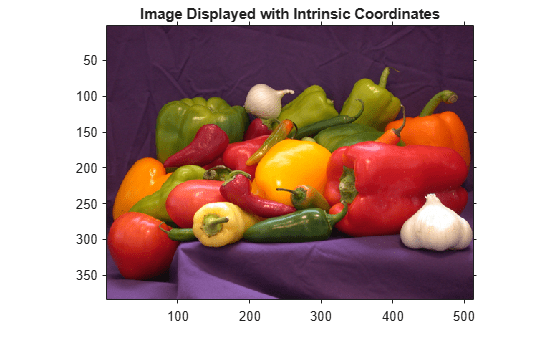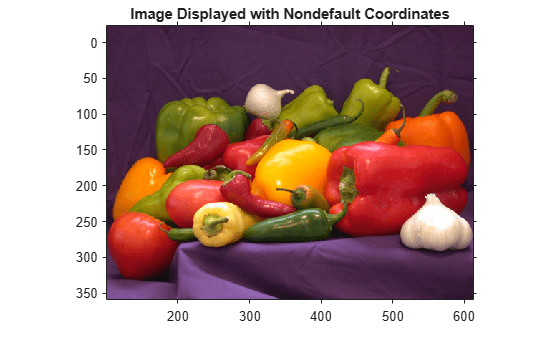## Define World Coordinates Using XData and YData Properties

To define a world coordinate system for an image, specify the `XData` and `YData` image properties for the image. The `XData` and `YData` image properties are two-element vectors that control the range of coordinates spanned by the image. When you do this, the MATLAB® axis coordinates become identical to the world (nondefault) coordinates. If you do not specify `XData` and `YData`, the axes coordinates are identical to the intrinsic coordinates of the image. By default, for an image `A`, `XData` is ```[1 size(A,2)]```, and `YData` is `[1 size(A,1)]`. With this default, the world coordinate system and intrinsic coordinate system coincide perfectly. (Another way to define a world coordinate system is to use spatial referencing—see Define World Coordinates Using Spatial Referencing.)

For example, if `A` is a 100 row by 200 column image, the default `XData` is [1 200], and the default `YData` is [1 100]. The values in these vectors are actually the coordinates for the center points of the first and last pixels (not the pixel edges), so the actual coordinate range spanned is slightly larger. For instance, if `XData` is [1 200], the interval in `X` spanned by the image is [0.5 200.5].

It is also possible to set `XData` or `YData` such that the x-axis or y-axis is reversed. You would do this by placing the larger value first. (For example, set the `YData` to [1000 1].) This is a common technique to use with geospatial data.

Several toolbox functions accept this `XData` and `YData` as arguments and return coordinates in the world coordinate system: `bwselect`, `imcrop`, `impixel`, `roipoly`, and `imtransform`.

### Display an Image using Nondefault Spatial Coordinates

Create an image of a 5-by-5 magic square.

`A = magic(5);`

Display this image with intrinsic coordinates. Here, the x- and y-coordinate ranges are both [1 5].

```image(A) axis image colormap(gca,jet(25)) title('Image Displayed with Intrinsic Coordinates');```Specify nondefault x- and y-coordinate ranges.

```x = [19.5 23.5]; y = [8.0 12.0];```

Display the image with the nondefault spatial coordinates using the `XData` and `YData` image properties.

```image(A,'XData',x,'YData',y) axis image colormap(gca,jet(25)) title('Image Displayed with Nondefault Coordinates');```